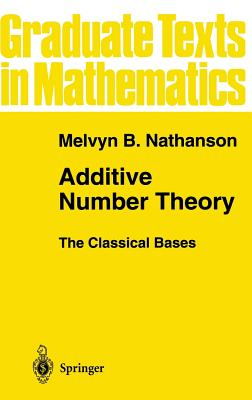Hardcover

List Price: 119.99*
* Individual store prices may vary.

Other Editions of This Title:
Paperback (11/19/2010)

Description

Hilbert's] style has not the terseness of many of our modem authors in mathematics, which is based on the assumption that printer's labor and paper are costly but the reader's effort and time are not. H. Weyl 143] The purpose of this book is to describe the classical problems in additive number theory and to introduce the circle method and the sieve method, which are the basic analytical and combinatorial tools used to attack these problems. This book is intended for students who want to lel?Ill additive number theory, not for experts who already know it. For this reason, proofs include many "unnecessary" and "obvious" steps; this is by design. The archetypical theorem in additive number theory is due to Lagrange: Every nonnegative integer is the sum of four squares. In general, the set A of nonnegative integers is called an additive basis of order h if every nonnegative integer can be written as the sum of h not necessarily distinct elements of A. Lagrange 's theorem is the statement that the squares are a basis of order four. The set A is called a basis offinite order if A is a basis of order h for some positive integer h. Additive number theory is in large part the study of bases of finite order. The classical bases are the squares, cubes, and higher powers; the polygonal numbers; and the prime numbers. The classical questions associated with these bases are Waring's problem and the Goldbach conjecture.

Springer, 9780387946566, 342pp.

Publication Date: June 25, 1996